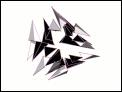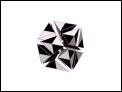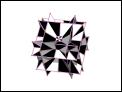Symmetry Tetrahedron Animations 8 images tree :: Geometry > Symmetry Tetrahedron Animations
In This Album:

 Image List Thumbnail Page
The Platonic solids can be divided into a number of congruent tetrahedra which share a common vertex at the centre of the solid. The maximum number can be made when the four vertices are the centres of the different dimensional elements of the solid: the centre of the solid itself; a face centre; an edge centre and a vertex (which is its own centre.)

The maximum number of tetrahedra is also the number of symmetries of the solid, and any symmetry of the solid maps the set of tetrahedra onto itself. The tetrahedra have no mirror symmetry.

The following animations rearrange the symmetry unit tetrahedra, sometimes grouped together, in various ways. The tetrahedra are coloured black and white according to their handedness. The animations preserve the rotational symmetry of the base polyhedron.

 thumbnails 1/1
 TetrahedronMed  Lrg
 TetrahedronMed  Lrg
 TetrahedronMed  Lrg
 CubeMed  Lrg
 CubeMed  Lrg
 CubeMed  Lrg
 DodecahedronMed  Lrg
 DodecahedronMed  Lrg
 1